# JP2009145305A - Rotation position detecting device - Google Patents

## Info

Publication number
JP2009145305A
JP2009145305A JP2007325920A JP2007325920A JP2009145305A JP 2009145305 A JP2009145305 A JP 2009145305A JP 2007325920 A JP2007325920 A JP 2007325920A JP 2007325920 A JP2007325920 A JP 2007325920A JP 2009145305 A JP2009145305 A JP 2009145305A
Authority
JP
Japan
Prior art keywords
angle
rotational
wave signal
theta
variable
Prior art date
Legal status (The legal status is an assumption and is not a legal conclusion. Google has not performed a legal analysis and makes no representation as to the accuracy of the status listed.)
Pending
Application number
JP2007325920A
Other languages
Japanese (ja)
Inventor
Shigeo Negi

Original Assignee
Nippon Yusoki Co Ltd

Priority date (The priority date is an assumption and is not a legal conclusion. Google has not performed a legal analysis and makes no representation as to the accuracy of the date listed.)
Filing date
Publication date
Application filed by Nippon Yusoki Co Ltd, 日本輸送機株式会社 filed Critical Nippon Yusoki Co Ltd
Priority to JP2007325920A priority Critical patent/JP2009145305A/en
Publication of JP2009145305A publication Critical patent/JP2009145305A/en
Pending legal-status Critical Current

• 238000007906 compression Methods 0.000 claims abstract description 39
• 235000020127 ayran Nutrition 0.000 claims abstract description 14
• 239000004794 expanded polystyrene Substances 0.000 claims description 14
• 230000007274 generation of a signal involved in cell-cell signaling Effects 0.000 claims description 4
• 230000000875 corresponding Effects 0.000 abstract description 11
• 238000010586 diagrams Methods 0.000 description 4
• 230000004048 modification Effects 0.000 description 2
• 238000006011 modification reactions Methods 0.000 description 2
• 280000306009 Every Angle companies 0.000 description 1
• 238000006243 chemical reactions Methods 0.000 description 1
• 239000004065 semiconductors Substances 0.000 description 1
• 230000001360 synchronised Effects 0.000 description 1

## Images

•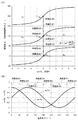•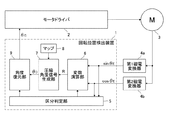•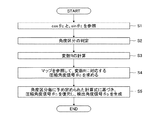•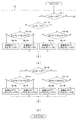•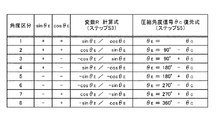•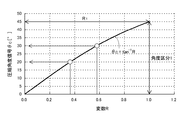•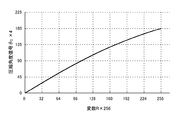•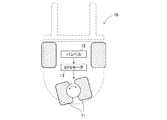•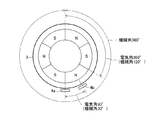## Abstract

<P>PROBLEM TO BE SOLVED: To provide a rotation position detecting device capable of highly precisely detecting the rotational position of a motor at low cost, using a comparably simple configuration. <P>SOLUTION: The rotational position detecting device 1 comprises a section determining part 5 for determining the angle sections belonging to a motor angle θ<SB>E</SB>as well as dividing all the angle range of the electrical angle θ<SB>E</SB>probable extent of all angle range into 8 angle sections of 45°, by using sine wave signals sinθ<SB>E</SB>and cosine wave signals cosθ<SB>E</SB>, varying according to the electrical angle θ<SB>E</SB>; a variable operation part 6 for calculating the variable R in the range of 0≤R<1 by using the sine wave signals sinθ<SB>E</SB>and cosine wave signals cosθ<SB>E</SB>; a compression angle signal generating part 7 for obtaining the compression angle signal θ<SB>C</SB>in the range of 0≤θ<SB>C</SB><45°, corresponding to the variable R using the relation "θ<SB>T</SB>=tan<SP>-1</SP>R" in the angle section "1"; and an angle restoring part 9 for generating detection angle signal θ<SB>D</SB>in the range of 0≤θ<SB>D</SB><360°, by restoring the compression signal θ<SB>C</SB>using a calculation formula predetermined for each angle section. <P>COPYRIGHT: (C)2009,JPO&INPIT

## Description

The present invention relates to a rotational position detection device that detects a rotational position of a motor using a sine wave signal and a cosine wave signal output from a pair of magnetoelectric conversion elements provided in the motor.

In general, in position control of a driven object using a motor, the rotational position of the motor is detected, and feedback control is performed according to the detection result. In order to accurately perform this position control, it is extremely important to improve the detection accuracy of the rotational position.
As is well known, the rotational position of the motor can be expressed using “mechanical angle” and “electrical angle”. However, in this specification, unless otherwise specified, the rotational position is expressed as “electrical angle θ E ”. Is expressed. The relationship between the mechanical angle and the electrical angle is as shown in FIG. For example, in the 6-pole motor 3, when the rotor makes one rotation with respect to the stator (= mechanical angle 360 °), the electrical angle changes by 1080 °. An electrical angle of 360 ° corresponds to a mechanical angle of 120 °.

As an example in which the position control is performed, in the cargo handling vehicle 10 illustrated in FIG. 8, the handle 12 is operated by a passenger, and a command value (electric signal) corresponding to the operation amount is input to the EPS motor 13. And the angle of the steering wheel 11 is controlled by the rotational operation of the EPS motor 13, and the advancing direction of the cargo handling vehicle 10 is determined.
If the rotational position of the EPS motor 13 cannot be accurately detected in the cargo handling vehicle 10, the relationship between the operation amount of the handle 12 and the angle of the steering wheel 11 may be shifted, or the EPS motor 13 may be caused by unnatural torque fluctuations. , The ride comfort of the passengers may deteriorate. The same applies to other traveling vehicles as well as cargo handling vehicles.

Therefore, various studies have been made for detecting the rotational position of the motor with high accuracy. For example, in the position control motor device described in Patent Document 1, a pair of Hall elements (4a, 4b) whose electrical angles are shifted by 90 ° are arranged in the vicinity of the motor 3 (see FIG. 9), and the electrical angle θ E sinusoidal signal sin [theta E and the cosine wave signal cos [theta] E changes according to which change as geometric.
The sinusoidal signal sin θ E in the range of 0 ° ≦ θ E <45 °, 135 ° ≦ θ E <225 °, 315 ° ≦ θ E <360 °, and 45 ° ≦ θ E <135 °, 225 ° ≦ A detection signal corresponding to the electrical angle θ E is generated by connecting the cosine wave signals cos θ E in the range of θ E <315 ° and treating them as straight lines.
JP-A-4-208090

However, since the sine wave signal sin θ E and the cosine wave signal cos θ E in the above range are not strictly straight lines, the rotational position of the motor cannot be detected with high accuracy by the generated detection signal (Patent Document 1). FIG. 3).
Therefore, even if the method in the position control motor device is applied to the cargo handling vehicle 10 shown in FIG. 8, the relationship between the operation amount of the handle 12 and the angle of the steering wheel 11 is still different, or the EPS motor 13 is not operated. There are cases where the ride comfort of the passengers deteriorates due to natural torque fluctuations.

Therefore, an object of the present invention is to provide a rotational position detection device that can detect the rotational position of a motor with high accuracy at a low cost with a relatively simple configuration.

In order to solve the above-described problem, a rotational position detection device according to the present invention includes:
A motor whose electrical angle θ E changes according to the relative rotational position of the rotor with respect to the stator is provided with a pair of magnetoelectric converters, and a sine wave signal sin θ E and a cosine wave signal cos θ output from the magnetoelectric converter. A rotational position detecting device that detects the rotational position based on E , i) whether or not the sine wave signal sin θ E and the cosine wave signal cos θ E are positive, and the absolute value of the sine wave signal sin θ E Based on the magnitude relationship between the value and the absolute value of the cosine wave signal cos θ E , the entire angle range that the electrical angle θ E can take is divided into a plurality of angle segments in increments of 45 °, and the electrical angle θ E said angular segment determining angular segment determination unit belonging, ii) the one of the absolute value of the absolute value and the cosine wave signal cos [theta] E of the sine wave signal sin [theta E, performs division divided by the larger the smaller, 0 A variable calculating section for calculating the variable R in the range R <1 of using the relationship iii) type "θ C = tan -1 R" , 0 ≦ θ C < compressed angle signal compressed in a range of 45 ° a compression angle signal generator for obtaining the theta C, iv) based on said angular segment predetermined calculation formula for each, to restore the compressed angle signal theta C in can take full angular range of the electrical angle theta E, an angle restoring unit for generating a detection angle signal theta D, characterized by comprising a.

Preferably, a calculation formula for the variable R is determined in advance for each angle segment.

More preferably, the rotational position detection device according to the present invention further comprises v) a map that holds the relationship between the variable R and the compression angle signal θ C , and each time the compression angle signal θ C is obtained, The compression angle signal θ C can be obtained without calculating “θ C = tan −1 R”.

Moreover, in order to solve the said subject, the traveling vehicle which concerns on this invention is the following.
i) any one of the rotational position detection devices described above, ii) an EPS motor in which the rotational position detection device detects an electrical angle θ E that changes according to the relative rotational position of the stator and the rotor, and iii) the steering wheel that is controlled by the EPS motor, iv) and a motor driver for driving the EPS motor, said motor driver, with reference to the detection angle signal theta D outputted from the rotational position detecting device, the The EPS motor is driven.

ADVANTAGE OF THE INVENTION According to this invention, the rotational position detection apparatus which can detect the rotational position of a motor with high precision at low cost by a comparatively simple structure can be provided.

First, the basic concept of the rotational position detection device according to the present invention will be described. Hereinafter, a case where the rotational position of the 6-pole motor is detected will be described.

[Basic concept]
As shown in FIG. 2, the rotational position detection device 1 according to the present invention includes a sine wave signal sin θ E output from the first magnetoelectric converter 4 a and a cosine wave signal cos θ E output from the second magnetoelectric converter 4 a. the rotational position of the motor 3 based on the bets, that is, detects the electrical angle theta E. The first magnetoelectric converter 4a and the second magnetoelectric converter 4b are provided in the vicinity of or inside the motor 3 with the electrical angle shifted by 90 ° (= mechanical angle 30 °) (see FIG. 9).

For on the basis of the two signals that the sine wave signal sin [theta E and the cosine wave signal cos [theta] E obtaining an electrical angle theta E, for example, collectively the two signals by performing a calculation such as "sinθ E / cosθ E" There is a need to. In FIG. 1A, tan θ E is calculated using the formula “sin θ E / cos θ E ” to determine the electrical angle θ E , but this has the following problems.
That is, according to the method shown in FIG. 1A, for example, when tan θ E = 0, it is impossible to specify whether the electrical angle θ E is 0 ° or 180 ° (first problem) ). Further, in the vicinity of the asymptote of electrical angle θ E = 90 °, 270 °, tan θ E becomes extremely large or small, which hinders calculation (second problem).

Therefore, in the rotational position detection device according to the present invention, the entire angle range that can be taken by the electrical angle θ E is divided into 8 angle sections in increments of 45 °, and the electrical angle θ E and the sine wave shown in FIG. From the relationship between the signal sin θ E and the cosine wave signal cos θ E , the angle classification to which the electrical angle θ E to be detected belongs is determined. As is clear from FIG. 1B, the determination of the angle division is performed by (1) positive / negative of the sine wave signal sin θ E , (2) positive / negative of the cosine wave signal cos θ E , and (3) sine wave signal sin θ E and cosine. This can be done by determining the magnitude relationship between the absolute values of the wave signal cos θ E.
For example, if | cos θ E |> | sin θ E |, sin θ E > 0, and cos θ E > 0, the angle division is “1”. In addition, in the case of | sin θ E |> | cos θ E |, sin θ E <0, and cos θ E <0, the angle division is “6”.
By determining the angle division, the first problem can be solved.

Further, in the rotational position detection device according to the present invention, the electrical angle in all angle segments is obtained using the region where the second problem cannot occur, that is, the relationship between tan θ E and the electrical angle θ E in the angle segment “1”. It is characterized in that θ E is specified.
Specifically, as shown in FIG. 1A, tan θ E in the angle section “1” is not less than 0 and can only take a value less than 1, and therefore, a variable that falls within this range is a sine wave signal sin θ E. must be generated from the cosine wave signal cos [theta] E. In the present invention, this variable is “variable R”, and the calculation is performed by dividing the smaller one of the absolute value of the sine wave signal sin θ E and the absolute value of the cosine wave signal cos θ E by the larger one.
Then, the compression angle signal θ C corresponding to the variable R is obtained using the relationship “θ C = tan −1 R” of the angle section “1”.

However, since the value that the variable R can take is 0 ≦ R <1, the angle that the compression angle signal θ C can take is 0 ≦ θ C <45 °, and information on the angle division is missing.
Therefore, in the rotational position detection device according to the present invention, the compression angle signal θ C is restored based on the predetermined angle division, and finally the detection angle signal θ D (0 ≦ 0) corresponding to the electrical angle θ E is obtained. θ D <360 °) can be obtained.

[Embodiment]
Next, preferred embodiments for realizing the basic concept of the present invention will be described with reference to the accompanying drawings.
2 is a block diagram showing the configuration of the rotational position detecting device according to the present invention, FIG. 3 is a flowchart showing the operation of the rotational position detecting device according to the present invention, and FIG. 4 is step S2 of the rotational position detecting device according to the present invention. FIG. 5 is a flow chart showing in detail the operation of FIG. 5, FIG. 5 is a table showing a calculation formula for a variable R predetermined for each angle segment, and a calculation formula for restoring the compression angle signal θ C , and FIG. 6 is a compression angle signal θ It is a graph which shows the map referred when calculating | requiring C.

In the block diagram shown in FIG. 2, the motor driver 2 is mainly composed of a power semiconductor element such as an IGBT and outputs a command value to the motor 3. The motor 3 is a three-phase synchronous motor, for example, and rotates upon receiving a voltage command value. The motor 3 includes a sensor magnet (not shown) that rotates with the rotor. The sensor magnet is sinusoidally magnetized according to the number of poles (for example, six poles) of the motor 3.

A first magnetoelectric converter 4a and a second magnetoelectric converter 4b are provided in the vicinity of the sensor magnet, and a change in the magnetic field according to the relative rotational position of the rotor with respect to the stator is output as an electrical signal. In the present embodiment, the first magnetoelectric converter 4a and the second magnetoelectric converter 4b are Hall elements having the same characteristics. As shown in FIG. 9, since the first magnetoelectric converter 4a and the second magnetoelectric converter 4b are arranged with the electrical angle shifted by 90 ° (= mechanical angle 30 °), the first magnetoelectric converter 4a When the output electric signal is a sine wave signal sin θ E , the electric signal output from the second magnetoelectric converter 4 b is a cosine wave signal cos θ E.

Normally, analog signals are output from the first magnetoelectric converter 4a and the second magnetoelectric converter 4b, but this signal format can be arbitrarily changed. For example, the sine wave signal sin θ E and the cosine wave signal cos θ E converted into digital signals by an A / D converter and converted into digital signals may be input to the rotational position detection device 1.

When the sine wave signal sin θ E and the cosine wave signal cos θ E are input to the rotational position detection device 1 (step S1 in FIG. 3), first, the section determination unit 5 determines the angle section to which the electrical angle θ E belongs. (Step S2).

Referring to FIG 4, in step S2, firstly, the sine wave signal sin [theta E whether it is positive is determined (step S2-1). If the sine wave signal sin [theta E is positive, angular segment will have been determined to be either "1" ~ "4". Subsequently, it is determined whether or not the cosine wave signal cos θ E is positive (step S2-2a). If the cosine wave signal cos θ E is also positive, it is determined that the angle segment is either “1” or “2”.
Subsequently, in step S2, it is determined whether or not the absolute value of the cosine wave signal cos θ E is larger than the absolute value of the sine wave signal sin θ E (step S2-3a). If this condition is satisfied, it is determined that the angle division is “1 (0 ≦ θ E <45 °)” (step S2-4a). On the other hand, when the condition of step S2-3a is not satisfied, it is determined that the angle division is “2 (45 ≦ θ E <90 °)” (step S2-4b).
Similarly, in other cases, it is determined to which angle section “3” to “8” the electrical angle θ E belongs.

After all, in step S2 shown in FIG. 4, (1) whether or not the sine wave signal sin θ E is positive, (2) whether or not the cosine wave signal cos θ E is positive, and (3) sine wave signal sin θ. The magnitude relationship between the absolute values of E and the cosine wave signal cos θ E is sequentially determined. Based on this determination result, angular segment including Electrical angle theta E is determined.

When the determination of the angle classification (step S2 in FIG. 3) by the classification determination unit 5 is completed, the variable R is calculated by the variable calculation unit 6 (step S3). This calculation, the sine wave signal sin [theta E output from the first magneto-electric transducer 4a, a cosine wave signal cos [theta] E output from the second magnetoelectric transducer 4b, and the determination result of category determination unit 5 is used The

As shown in FIG. 5, the calculation of the variable R in the present embodiment is performed based on a calculation formula determined in advance for each angle segment. In each calculation formula, of the sine wave signal sin θ E and the cosine wave signal cos θ E , the larger absolute value is the denominator and the smaller one is the numerator. Further, the sine wave signal sin θ E and the cosine wave signal cos θ E are appropriately converted to positive values by adding a sign “−”. This ensures that the variable R is greater than or equal to 0 and less than 1.

Specifically, when the angle section is “1”, the variable R is calculated by the expression “sin θ E / cos θ E ”. As is clear from FIG. 1B, in the angle section “1”, | cos θ E |> | sin θ E |, sin θ E > 0, and cos θ E > 0 are satisfied, and therefore the value that the variable R can take Is 0 ≦ R <1. When the angle section is “6”, the variable R is calculated by the expression “−cos θ E / −sin θ E ”. In the angle section “6”, | sin θ E |> | cos θ E |, sin θ E <0, and cos θ E <0 are satisfied. In this case, the possible value of the variable R is 0 ≦ R <1. .

When the calculation of the variable R by the variable calculation unit 6 (step S3 in FIG. 3) ends, the compression angle signal θ C is obtained by the compression angle signal generation unit 7 (step S4). For this, the map 8 and the variable R calculated by the variable calculation unit 6 are used.
As shown in FIG. 6, the map 8 holds the relationship “θ C = tan −1 R” between the variable R of the angle section “1” and the compression angle signal θ C shown in FIG. According to the map 8, the compression angle signal θ C (0 ≦ θ C <45 °) corresponding to the variable R can be obtained. Instead of using the map 8, “θ C = tan −1 R” may be calculated every time the compression angle signal θ C is obtained.

Specifically, when the electrical angle θ E to be detected is 30 ° (sin θ E = 0.500, cos θ E = 0.866), it is determined in step S2 that the angle division is “1”, and step S3 The variable R (= sin θ E / cos θ E ) is calculated as 0.577. Then, the compression angle signal θ C (= 30 °) corresponding to the variable R of 0.577 is obtained from the map of FIG.
If the electrical angle θ E to be detected is 60 ° (sin θ E = 0.866, cos θ E = 0.500), it is determined in step S2 that the angle segment is “2”, and the variable R in step S3. (= Cos θ E / sin θ E ) is calculated as 0.577. Then, the compression angle signal θ C (= 30 °) corresponding to the variable R of 0.577 is obtained from the map of FIG. That is, when the electrical angle theta E to be detected in the 30 ° and 60 °, both the compression angle signal theta C becomes 30 °.

More specifically, when the electrical angle θ E to be detected is 110 ° (sin θ E = 0.940, cos θ E = −0.342), it is determined in step S2 that the angle division is “3”. In step S3, the variable R (= −cos θ E / sin θ E ) is calculated as 0.364. Then, the compression angle signal θ C (= 20 °) corresponding to the variable R of 0.364 is obtained from the map of FIG.
If the electrical angle θ E to be detected is 250 ° (sin θ E = −0.940, cos θ E = −0.342), it is determined in step S2 that the angle section is “6”, and in step S3. The variable R (= −cos θ E / −sin θ E ) is calculated as 0.364. Then, the compression angle signal θ C (= 20 °) corresponding to the variable R of 0.364 is obtained from the map of FIG. That is, even when the electrical angle theta E to be detected in the 110 ° and 250 °, both the compression angle signal theta C becomes 20 °.

When the compression angle signal θ C is obtained, the angle restoration unit 9 subsequently restores the compression angle signal θ C (step S5 in FIG. 3). This restoration is performed based on a calculation formula (see FIG. 5) determined for each angle section with reference to the determination result in the section determination unit 5.

That is, when the electrical angle θ E to be detected is 60 ° (angle section “2”), the restoration is performed by the expression “90−θ C ”. As described above, the compression angle signal theta C is because it is 30 °, after all, the detection angle signal theta D becomes 60 °.
In the case of an electrical angle theta E is 110 ° to be detected (an angle indicator "3") is performed restored by the formula "90 + θ C". As described above, the compression angle signal theta C is because it is 20 °, after all, the detection angle signal theta D becomes 110 °.
Further, when the electrical angle θ E to be detected is 250 ° (angle section “6”), the restoration is performed by the expression “270−θ C ”. As described above, the compression angle signal theta C is because it is 20 °, after all, the detection angle signal theta D becomes 250 °.
In the case of an electrical angle theta E is 30 ° to be detected (an angle indicator "1"), there is no particular need to perform recovery, detection angle signal theta D remains 30 °.

With reference to FIG. 2, the detected angle signal θ D generated by the angle restoration unit 9 is output to the motor driver 2. The motor driver 2 compares the electrical angle theta E of the motor 3 as a target and the detection angle signal theta D, if there is an error, and outputs the command value to correct the error in the motor 3 .

As described above, according to the rotational position detecting device according to the present invention, it based on the sine wave signal sin [theta E and the cosine wave signal cos [theta] E, obtain detected angle signal theta D corresponding to an electrical angle theta E to be detected Can do. Further, in the rotational position detection apparatus according to the present invention, since complicated calculation is omitted using the map shown in FIG. 6, it is not necessary to perform advanced calculation processing, and the electrical angle is reduced with a simple configuration. θ E can be detected.

Note that the rotational position detection device according to the present invention is not limited to the above configuration, and various modifications can be considered.
For example, when the value that can be handled by the compression angle signal generation unit 7 is limited to a positive integer, the detection accuracy can be improved by using the map shown in FIG. That is, in the map shown in FIG. 7, the horizontal axis is a value obtained by multiplying "256" to the variable R, the vertical axis represents the value obtained by multiplying the "4" to the compression angle signal theta C. This makes it possible to handle, separated 45 ° to 180 steps, it is possible to detect the electrical angle theta E with high accuracy of 0.25 °.

Further, the applicable range of the rotational position detection device according to the present invention is very wide, and can be applied to all fields where it is necessary to detect the rotational position of the motor.

It is a basic concept of the rotational position detection device according to the present invention, (A) is a graph showing the relationship between tan θ E and electrical angle θ E , and the relationship between variable R and compression angle signal θ C in angle section “1”; (B) is a graph showing the relationship between the electrical angle theta E and the sine wave signal sin [theta E and the cosine wave signal cos [theta] E. It is a block diagram which shows the structure of the rotational position detection apparatus which concerns on this invention. It is a flowchart which shows the whole operation | movement of the rotational position detection apparatus which concerns on this invention. It is a flowchart explaining in detail step S2 in the operation of the rotational position detection device according to the present invention. It is a table | surface which shows the calculation formula for the variable R predetermined for every angle division, and the calculation formula for decompress | restoring compression angle signal (theta) C. It is a graph which shows the map referred in step S5. It is a graph which shows the modification of the map referred in step S5. It is a block diagram explaining the composition of a cargo handling vehicle simply. It is a schematic cross section of a 6 pole motor for demonstrating the relationship between an electrical angle and a mechanical angle.

Explanation of symbols

DESCRIPTION OF SYMBOLS 1 Rotation position detection apparatus 2 Motor driver 3 Motor 4a 1st magnetoelectric converter 4b 2nd magnetoelectric converter 5 Classification determination part 6 Variable calculation part 7 Compression angle signal generation part 8 Map 9 Angle restoration part 10 Cargo vehicle 11 Steering wheel 12 Steering wheel 13 EPS Motor R Variable

## Claims (4)

1. A motor whose electrical angle θ E changes according to the relative rotational position of the rotor with respect to the stator is provided with a pair of magnetoelectric converters, and a sine wave signal sin θ E and a cosine wave signal cos θ output from the magnetoelectric converter. A rotational position detecting device for detecting the rotational position based on E ,
i) Based on whether or not the sine wave signal sin θ E and the cosine wave signal cos θ E are positive, and the magnitude relationship between the absolute value of the sine wave signal sin θ E and the absolute value of the cosine wave signal cos θ E , with dividing the possible total angle range of said electrical angle theta E into a plurality of angular segment in increments of 45 °, and determines an angular segment determination unit said angular segment belonging of said electrical angle theta E,
Among the absolute value of the absolute value and the cosine wave signal cos [theta] E of ii) the sine wave signal sin [theta E, performs division divided by the larger the smaller, to calculate the variable R in the range 0 ≦ R <1 variable An arithmetic unit;
iii) a compression angle signal generation unit for obtaining a compression angle signal θ C compressed in a range of 0 ≦ θ C <45 ° using the relationship of the expression “θ C = tan −1 R”;
iv) An angle restoration unit that restores the compression angle signal θ C to an entire angle range that the electrical angle θ E can take based on a calculation formula predetermined for each angle section, and generates a detection angle signal θ D When,
A rotational position detecting device comprising:
2.   The rotational position detection apparatus according to claim 1, wherein a calculation formula of the variable R is determined in advance for each angle section.
3. v) a map that holds the relationship between the variable R and the compression angle signal θ C ;
Further comprising
Every time of determining the compression angle signal theta C, rotation according to claim 1 without performing calculation of the formula "θ C = tan -1 R" , wherein the can determine the compression angle signal theta C Position detection device.
4. i) the rotational position detection device according to any one of claims 1 to 3;
ii) an EPS motor in which an electrical angle θ E that changes according to the relative rotational position of the stator and the rotor is detected by the rotational position detection device;
iii) a steered wheel controlled by the EPS motor;
iv) a motor driver for driving the EPS motor;
With
The motor driver, with reference to the detection angle signal theta D outputted from the rotational position detecting device, the traveling vehicle and drives the EPS motor.
JP2007325920A 2007-12-18 2007-12-18 Rotation position detecting device Pending JP2009145305A (en)

## Priority Applications (1)

Application Number Priority Date Filing Date Title
JP2007325920A JP2009145305A (en) 2007-12-18 2007-12-18 Rotation position detecting device

## Applications Claiming Priority (1)

Application Number Priority Date Filing Date Title
JP2007325920A JP2009145305A (en) 2007-12-18 2007-12-18 Rotation position detecting device

## Publications (1)

Publication Number Publication Date
JP2009145305A true JP2009145305A (en) 2009-07-02

# Family

## Family Applications (1)

Application Number Title Priority Date Filing Date
JP2007325920A Pending JP2009145305A (en) 2007-12-18 2007-12-18 Rotation position detecting device

## Country Status (1)

JP (1) JP2009145305A (en)

## Cited By (2)

* Cited by examiner, † Cited by third party
Publication number Priority date Publication date Assignee Title
US8204641B2 (en) 2009-07-31 2012-06-19 Denso Corporation Traction motor control apparatus for vehicle
US8487563B2 (en) 2009-11-27 2013-07-16 Denso Corporation Drive motor control apparatus for vehicle, motor control system, method for correcting rotation angle of motor, program for performing the same, rotation detecting apparatus

## Cited By (2)

* Cited by examiner, † Cited by third party
Publication number Priority date Publication date Assignee Title
US8204641B2 (en) 2009-07-31 2012-06-19 Denso Corporation Traction motor control apparatus for vehicle
US8487563B2 (en) 2009-11-27 2013-07-16 Denso Corporation Drive motor control apparatus for vehicle, motor control system, method for correcting rotation angle of motor, program for performing the same, rotation detecting apparatus

## Similar Documents

Publication Publication Date Title
US9540037B2 (en) Malfunction detection device for resolver
CN102549386B (en) For the method for the signal of analytic angle sensor
US8185342B2 (en) Estimating rotor angular position and velocity and verifying accuracy of position sensor outputs
US6707265B2 (en) Rotor angle detecting apparatus for DC brushless motors
JP5652434B2 (en) Motor control device and electric power steering device using the same
CN104052361B (en) Electric machine control system to compensate torque pulsation
Akrad et al. Design of a fault-tolerant controller based on observers for a PMSM drive
US9255817B2 (en) Rotation-angle detection device, image processing apparatus, and rotation-angle detection method
CN100417005C (en) Method of estimating magnetic pole position in synchronous motor
JP5679136B2 (en) Rotation angle detection device and electric power steering device provided with rotation angle detection device
CN104022708B (en) Electric variable-pitch driving system by speed sensorless technology and method thereof
JP4909797B2 (en) Motor control device
Kim Analysis on the charateristics of variable reluctance resolver considering uneven magnetic fields
US7548038B2 (en) Controller for motor
US6763622B2 (en) Amplitude detection method and apparatus for high frequency impedance tracking sensorless algorithm
US8872449B2 (en) Distributed-arrangement linear motor and control method of distributed-arrangement linear motor
JP2004201487A (en) Motor and its drive controlling apparatus
DE112010001309T5 (en) Drive control device for an electric motor
CN103066756B (en) A kind of electrical motor or electrical generator
JP5490601B2 (en) Method for determining the position of the magnetic flux vector of a motor
US7664619B2 (en) Fault detection unit for rotation angle detecting device
US8471506B2 (en) Motor control apparatus
CN103917851B (en) Error frequency component acquisition device, anglec of rotation acquisition device, motor control assembly and anglec of rotation acquisition methods
US9077278B2 (en) AC motor control apparatus
JP3546817B2 (en) Magnetic pole position detection device for motor

## Legal Events

Date Code Title Description
A521 Written amendment

Free format text: JAPANESE INTERMEDIATE CODE: A523

Effective date: 20090327

A131 Notification of reasons for refusal

Free format text: JAPANESE INTERMEDIATE CODE: A131

Effective date: 20090422

A02 Decision of refusal

Free format text: JAPANESE INTERMEDIATE CODE: A02

Effective date: 20090902